Storage Elements in Circuits
Capacitors and Inductors

 Outline: Capacitor Review Inductor Review DC Steady State Switches Analysis of circuits with switches and storage elements

Capacitor Review

A Capacitor is an element which stores charge. It is comprised of two
conducting plates sepparated by a non-conducting material called a dielectric.

For every + unit charge put on one plate, there is an equal - unit charge on the
the other plate. Thus the entire capacitor is charge neutral. Since the + and - charges are
separated by a dielectric there is an electric field (or voltage) across the capacitor.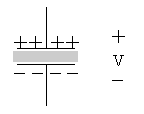If positive charge is put on one plate then work is done because like-charges repel each other.
Recall that voltage is related to work done on charge by the equation V=J/C.

The more charge on the capacitor's plates, the more work had to be done to put the charge there,
and the higher the voltage across the capacitor.

In this class all capacitors will be considered linear and will obey the following
charge-voltage relationship:

### q = CVIf this equation is differentiated we get:dq/dt = C(dV/dt) or      i = C(dV/dt)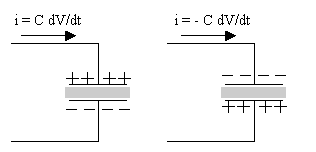The capacitor on the left is charging, therefore the voltage is increasing.
The capacitor on the right is actually discharging, therefore the voltage is decreasing.
Note the sign difference.

If voltage is not changing then dV/dt=0 and i=0. So in static (unchanging conditions)
the capacitor behaves like an 'open circuit' since no current flows.

Charge on a capacitor can not instantly leave. It takes some finite amount of time
for the charge to leave the plate. Therefore charge on the capacitor is continuous.
Since V = q/C then voltage across a capacitor is also continuous.
(i.e. The voltage across a capacitor can never change instantaneously.)

However the current can change instantaneously since i = C dV/dt.
This is because current is the rate of charge moving over time.

Energy stored in a capacitor is:
E = 1/2 CV2

### Using the above concepts, let's analyze the following circuit:

This circuit has both a switch and a capacitor:
The switch opens at t=0
The switch is closed for t<0 and is open for t>0.
This can be seen by inspecting the switch's arrow direction.
We will not try to analyze the circuit at t=0 since the circuit's
state at t=0 is unkonwn.
Instead we will look at the circuit at t=0- (the time right before
the switch moves) and t=0+ (the time right after the switch moves).

In this problem it is given that V4 = 8V at t=0-.

With this information find the following things at t=0- and t=0+:
1. The voltage across the capacitor.
2. The current i1
3. The current in the capacitor (going down)
4. dV/dt in the capacitor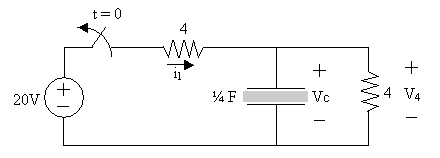Here are snapshots of the circuit at t=0- and t=0+:
_______________________________________________________

### t = 0-:

At t=0- the switch is closed. Since V4 = 8V and
and the capacitor is in parallel with V4, then VC = 8V at t=0-.

To determine i1 we need to find the voltage across the
horizontal 4 ohm resistor. To find this, we will apply KVL:
-20V + 4i1 + 8V = 0
4i1 = 20 - 8 = 12V
i1 = 12/4 = 3A

To find the current in the capacitor we have to apply KCL at the marked node:
i1 = i + iC
3A = 2A + iC
iC = 1A

dV/dt = iC/C = 1A/(.25F) = 4V/s
_______________________________________________________

### t = 0+:

At t=0+ the switch is open. Since the voltage across a capacitor can not
change instantly, then VC(0-) = VC(0+)
Therefore VC(0+) = 8V

Since the switch is now open no current flows through the horizontal resistor,
so i1 = 0

To find the current in the capacitor we have to apply KCL at the marked node:
i1 = i + iC
0A = 2A + iC
iC = -2A

dV/dt = iC/C = -2A/(.25F) = -8V/s

dV/dt is negative, therefore for t>0 the capacitor is discharging.
The energy stored in the capacitor is being absorbed by the resistor.
Eventually all the initial energy stored in the capacitor will be absorbed
by the resitor.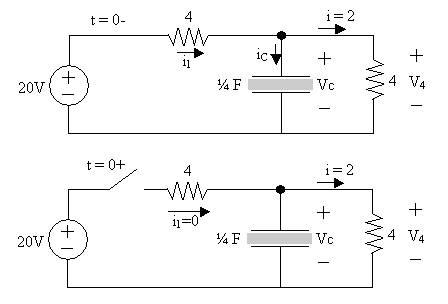## Study Problems

After clicking on the following link enter 6-1 for the problem and 1 for the step:
Study Problem 6-1

Top of Page

Inductor Review

An inductor is an element which stores a magnetic field. An inductor is
a wire coiled around a material called a core. The core is typically made of
a magnetic material however the core can be anything from a toilet paper roll to a piece of wood.
(The behavior of the inductor IS effected by the core material though.)

Recall from Physics that static charge sets up an electrostatic force. Also recall that
moving charge sets up a magnetic field called flux around the current-carrying wire.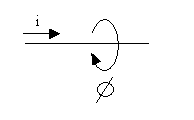If the wire is then coiled as shown below, then the flux increases linearly with every coil turn.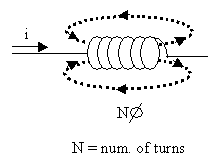The flux is proportional to the current flowing through the inductor.
We can use the following equation where L is the inductance of the inductor.(L is constant)

Flux = Li

Through experimentation it was found that if the flux changes then a voltage is induced.
If the flux is constant then there is no voltage across the inductor.

V = d(Flux)/dt
V = d(Li)/dt

V = L di/dt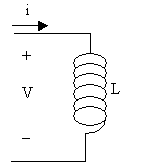When the current is changing a voltage is induced across the inductor.
This voltage opposes the change in the current.
When the current stops changing and stays constant, then the voltage collapses.
Therefore when conditions are constant (unchanging) the inductor appears like a
wire or short circuit.

Current is continuous in an inductor. In other words, current can not
change instantaneously. If current could change instantaneously then an infinite
voltage would be induced across the capacitor. Infinite voltage is a practical impossibility.

Therefore current can not change instantaneously through an inductor.
Voltage across an inductor can change instantaneously.

The energy stored in an inductor is:

E = 1/2 Li2